# My experience with Domain-Driven Design part 3: model schema

In this blog post I scream into the void about working in a project heavily influenced by Domain-Driven Design principles.

Previous editions:

## Context

• I’m a machine learning engineer at an edutech startup
• We are building a new machine learning model platform infrastructure
• Our architecture is heavily inspired by Domain-Driven Design and I want to share my lessons learned

## Final end result

This is the end result we are working towards.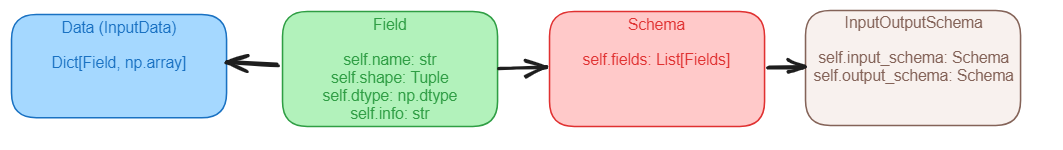It consists of:

• Field: Name and metadata of a feature
• Data: Data for the feature
• Schema: A list of fields
• InputOutputSchema: A collection of an input and output schema

## Model Schema

In this blog I want to talk about the idea of a model schema. Simply put, a model schema defines what comes in and what comes out of a model.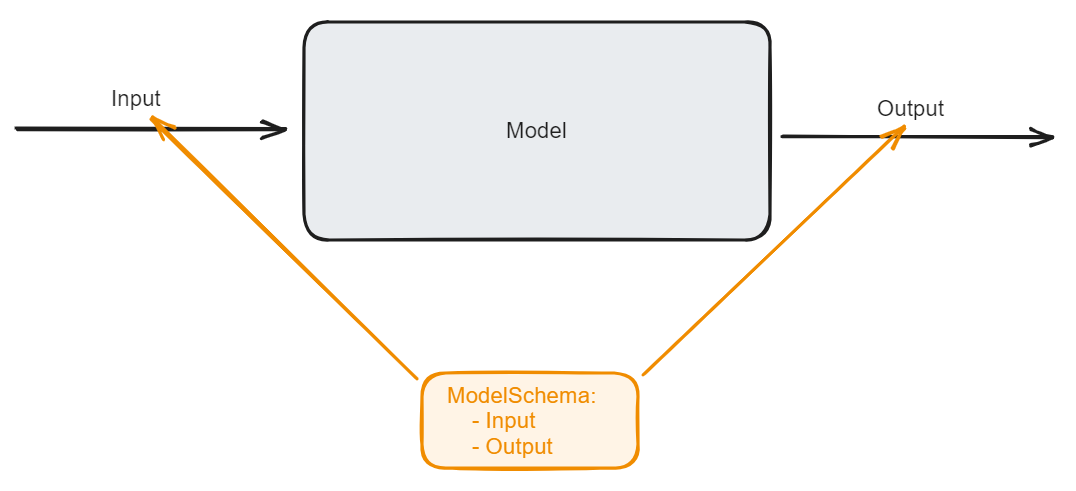But why do we need a model schema anyway?

For us, the model schema solves the problem of being able to pin down what exactly goes in and out of a model. When you have one model this problem is not so big, but once you start running multiple machine learning models in production next to each other tackling this problem early on (with model schemas) can save you a lot of headache down the line.

## Field

Where to start? We start our domain model with the definition of a “Field”. Every feature that we use is represented by its name (Field) and some data that comes with it (Data later). This field represents the metadata of the feature that we use and the field name is how we are going to refer to each feature. If we draw it it looks something like this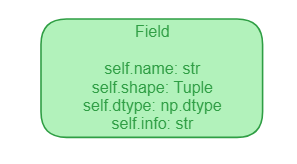In code, we create a dataclass with the right attributes

@dataclass(frozen=True, eq=True)
class Field:
name: str
dtype: np.dtype
shape: tuple
info: str


For example, consider a simple feature that represents the answer id. This is what it would look like:

# models/domain/fields.py
dtype=np.dtype(str),
shape=(-1,),  # (Batch_size,)
)


Of course, just having a field is not enough, a field without some data is useless. If the field (Field) is the name of the feature, then our data (Data) domain model object contains the actual data. Data is a dictionary that consists of fields as keys and numpy arrays (or tensors) as values.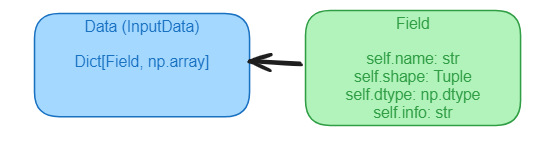A nice thing that we can do (already) with this domain terminology of just fields and data (over just a dataframe) is that we can slice a feature using its name instead of the index of the feature. This is much safer and less error-prone.

Having defined data and fields we can now define what a schema is.

## A schema is a list of fields

Now that we have a better and more precise naming for the features in our model, we can start discussing what a schema is. In our domain language a schema is just a list of fields. That’s it, nothing more. A schema is a list of fields.

Let’s draw the schema in relation to our fields domain model object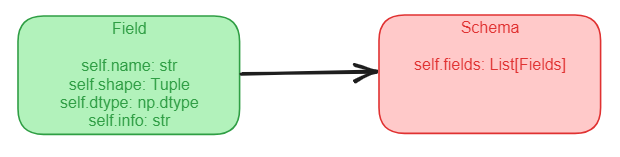In code, a Schema is another dataclass

@dataclass(frozen=True, eq=True)
class Schema:
fields: List[Field]
"""
A data class representing a schema of fields.  This can be the schema of a
dataset, the schema of model input_data features etc.
"""


For example, let’s define a simple schema consisting of two fields: the pupil id and the exercise id

from models.domain.schema import Schema
from models.domain.fields import PUPIL_ID, EXERCISE_ID

input_schema = Schema(fields=[PUPIL_ID, EXERCISE_ID])


Now finally, with schemas defined we can talk about what we are really interested in: the input/output schema.

## The input/output schema

After having defined fields and schemas, the extension to an input output schema is very natural. An input/output schema is a collection of an input schema and an output schema.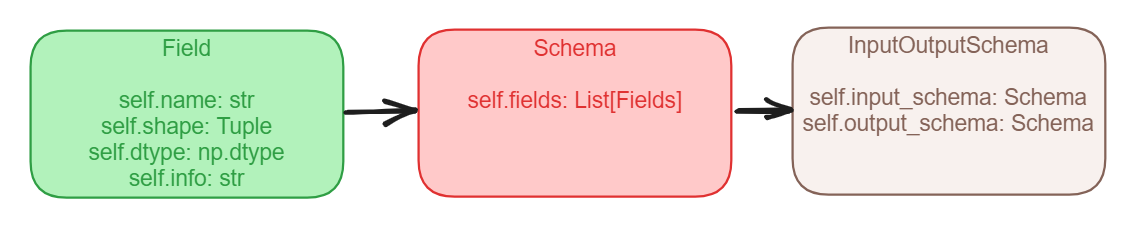In code this is another dataclass

@dataclass(frozen=True, eq=True)
class InputOutputSchema:
input_schema: Schema
output_schema: Schema
"""
A data class representing the input_data and output fields of a data transformer, model etc.
"""


For example, imagine we have a model that has some function get_io_schema() that returns the input/output schema. For this particular model the schema has as inputs the exercise id and pupil id and as outputs predictions per exercise id.

class Model (TorchModelInterface):
...
def get_io_schema(self) -> InputOutputSchema:
"""Returns the input/output schema"""
schema = InputOutputSchema.from_fields(
input_fields=[EXERCISE_ID, PUPIL_ID],
output_fields=[EXERCISE_ID_PREDICTION],
)
return schema



The nice thing is that we never have to worry about data integrity anymore as we can quickly compare the schema of the model and data, and check whether the data schema has all the columns that the model expects!

assert model.get_io_schema().is_in(dataset.get_schema())


# Wrapping upIn this blog post I talked about the concept of a model schema. A model schema specifies what goes in and what comes out of a model. This provides an intuitive and scalable solution to the problem of data definitions. It takes a bit more work up-front but it leads to a very consistent data API.

To summarise, this is our domain model for the schema:

• Data, which is a dictionary with fields as keys and some data as values
• A schema, which is a list of fields
• An input/output schema, which is a collection of two schemas (input and output)

Tags:

Categories:

Updated: